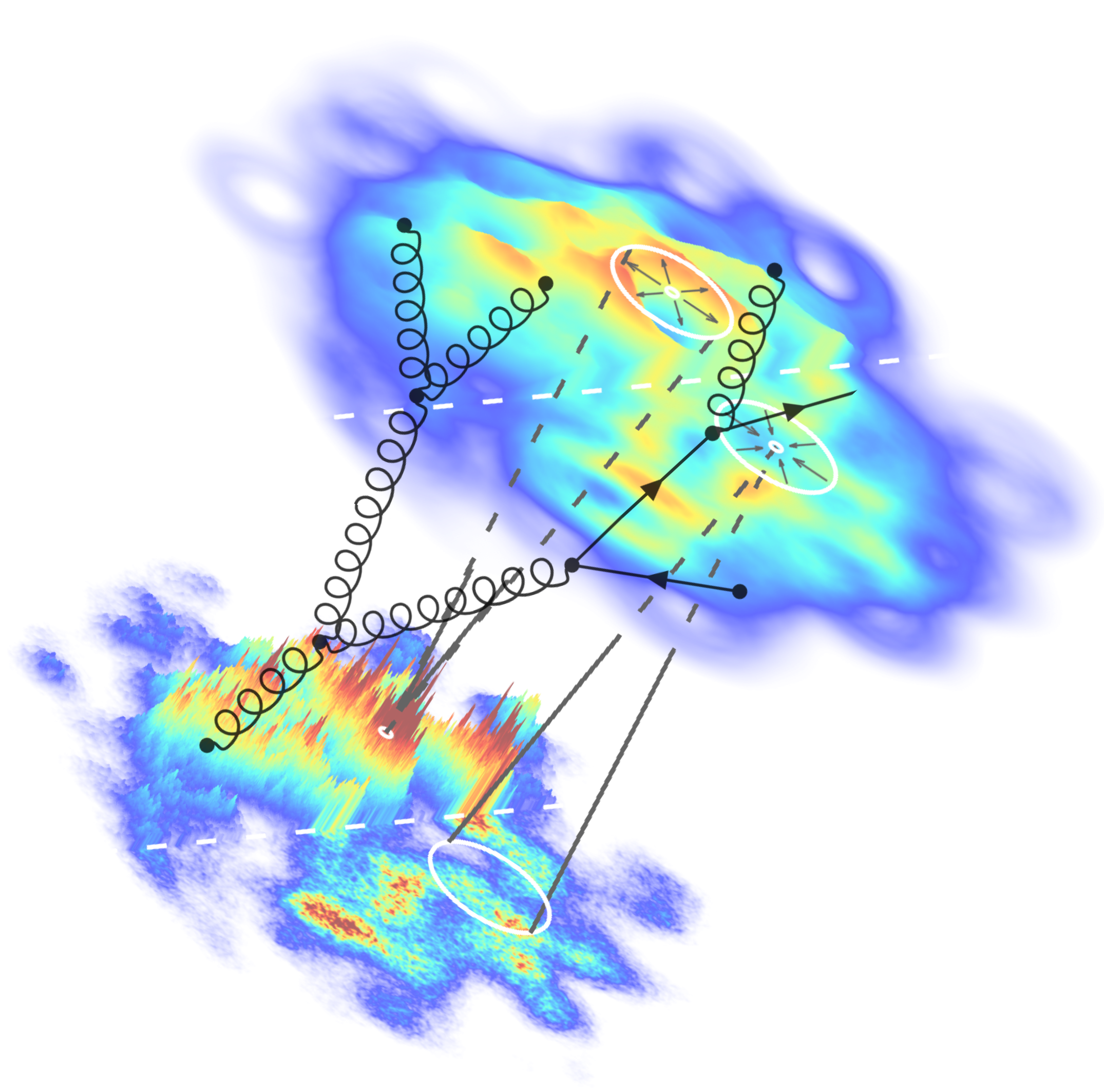# NON-EQUILIBRIUM PHYSICS WS 18/19

Lectures: Mon,Wed 12:00-14:00 D6-135
Tutorials: Fri 14:00-16:00 U2-232

Instructor: Jun.-Prof. Dr. Soeren Schlichting
(sschlichting AT physik.uni-bielefeld.de)

Tutor: Dennis Schroeder
(dennis AT physik.uni-bielefeld.de)

This graduate (M.Sc.) level course provides an introduction to non-equilibrium statistical physics, based on the book of N. Pottier on ``Nonequilibrium Statistical Physics``

Topics include:

- Thermodynamics of irreversible processes
- Statistical description of non-eq. systems
- Kinetic theory
- Weak wave turbulence
- Stochastic processes
- Linear repsonse theory

Course materials and exercise sheets will become available below over the course of the semester.

Course evaluation for Lectures and Tutorials .

Exercise sheets:

Exercise Sheet 1 -- Oct 12th

Exercise Sheet 2 -- Oct 19th

Exercise Sheet 3 -- Oct 26th

Exercise Sheet 4 -- Nov 2nd

Exercise Sheet 5 -- Nov 16th

Exercise Sheet 6 -- Nov 23rd

Exercise Sheet 7 -- Nov 30th

Exercise Sheet 8 -- Dec 7th

Exercise Sheet 9 -- Dec 14th

Exercise Sheet 10 -- Dec 21st

Exercise Sheet 11 -- Jan 11th

Exercise Sheet 12 -- Jan 18th

Exercise Sheet 13 -- Jan 25th

Exercise Sheet 14 -- Feb 1st

Course materials:

Week 1 -- Thermodynamics of irreversible processes: Equilibrium thermodynamics (reminder), Description of irreversible processes, Local equilibrium, Entropy production in discrete & continuous systems
Notes from Oct 8th and Notes from Oct 10th

Week 2 -- Thermodynamics of irreversible processes: Linear irreversible proccesses, Simple transport phenomena, Curie and Onsager relations
Notes from Oct 15th

Week 3 -- Thermodynamics of irreversible processes: Coupled transport phenomena, Dynamics of simple fluids, Navier-Stokes equation
Notes from Oct 22th and Notes from Oct 24th

Week 4 -- Thermodynamics of irreversible processes: Simple problems in fluid dynamics
-- Statistical description of non-equilibrium systems: Description of classical & quantum systems
Notes from Oct 22th and Notes from Oct 24th

Week 5 -- Statistical description of non-equilibrium systems: Wigner-Weyl formalism
Notes from Nov 5th and Nov 7th

Some additional LaTeX Notes on Wigner-Weyl formalism

Week 6 -- Statistical description of non-equilibrium systems: Non-equilibrium path integral, reduced phase-space distributions
Notes from Nov 12th and Notes from Nov 14th

Some additional LaTeX Notes on non-equilibrium path integral

Week 7 -- Statistical description of non-equilibrium systems: BBGKY Hierarchy, Boltzmann equation
Notes from Nov 19th and Notes from Nov 21st

Week 8 -- Kinetic theory/Boltzmann equation: Balance equations, Statistical entropy, H-Theorem
Notes from Nov 26th and Notes from Nov 28th

Week 9 -- Kinetic theory/Boltzmann equation: Equilibrium solutions, Hilbert expansion, Relaxation Time Approximation
Notes from Dec 3rd and Notes from Dec 5th

Week 10 -- Kinetic theory/Boltzmann equation: Calculation of transport coefficients, Hydrodynamic limit
Notes from Dec 10th and Notes from Dec 12th

Week 11 -- Kinetic theory/Boltzmann equation: Hydrodynamic limit
Notes from Dec 17th
-- Wave turbulence: Stationary turbulence, Richardson cascade, Kolmogorov-Zhakarov spectra Notes from Dec 19th
See also textbook V.E. Zakharov, V.S. L'vov and G. Falkovich; Kolmogorov Spectra of Turbulence I: Wave turbulence (Chapters 1,2,3)

Week 12 -- Stationary turbulence: Kolmogorov-Zhakarov spectra, Energy cascade, Decaying turbulence
Notes from Jan 7th and Notes from Jan 9th